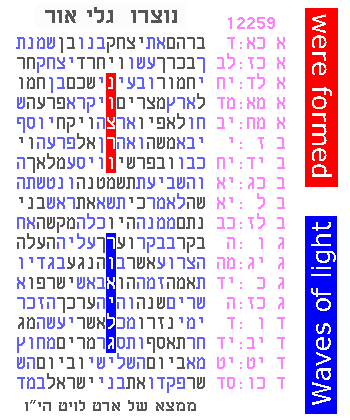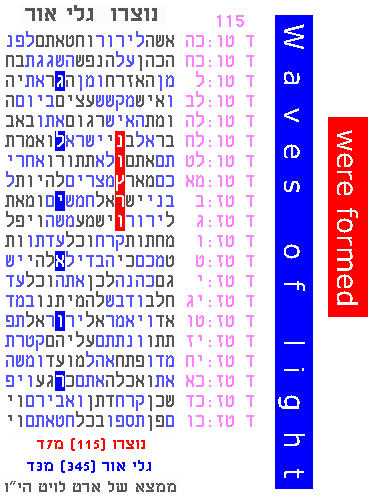Essential Introduction - Measuring the significance
 Summary Introduction/ Evidence Evidence Newest Motivations as of 2007 as of 2010 EvidenceHOME   PARTICIPATEMeasuring significance

We calculate the significance of one or more ELSs being located near other ELSs, using specific procedures, called protocols, run by computer. The protocols measure the Torah meetings by comparing them to monkey texts (non-Torah texts where we expect no codes). That is, the measure of how seldom we see similar kinds of ELS meetings in monkey texts is the estimate of probability for the Torah text.

Our protocols follow the rigorous techniques described and developed by Professor Robert M. Haralick. The protocols that we increasingly use (since 2004), differ only in their treatment of related words near the axis (central ELS). Related key words are freely searched in the area of the axis (rather than limiting the search to small-skip instances of the related words; this is actually an option in Professor Haralick's software, called linking, but we built this into our software as a permanent feature). We can thereby measure most kinds of codes using the following vertical, horizontal, and mixed variations.

Vertical ("one-column") protocol

In the following code we have ELSs for "waves of light" and "were formed/created" appearing in a one-column arrangment near each other.The one-column protocol examines the monkey texts as follows. It determines that these two ELSs appear in a single column "competitively" only 10 times in 12,000 monkey text trials. This yields a p-level (like a probability) of .00083, or 1 in 1200. "Competitive" means that the monkey text's arrangement has a more impressive combination of proximity and skip than the Torah's arrangement.

Sanity check

It is often possible to separately estimate the significance, as a "sanity check". Sometimes this check is simple enough to do with high school mathematics, as explained here.

Horizontal protocol

In addition to the one-column protocol described above, which handles strictly vertical arrangements, we use a 1D protocol to measure clusters of ELSs that occur in very short segments of the text - often pictured horizontally. For example, here is a second significant find for the same key words as above, this time in a 1D arrangement (and it has a similar significance):Axis-based (horizontal and vertical mixed) protocols

We handle parallel, vertical ELSs (optionally mixed with horizontal ELSs), based on a starting key word which acts as an "axis". We use a fixed axis-based protocol in cases where we have reason to focus on a particular vertical ELS - we will see many such cases where we have a "pointer" to a particular location in the text - i.e. a reason to search there. We use a floating axis-based protocol in cases like the following example (yet again using the same key words as above). Here we have no reason to prefer one particular location of the text:In each protocol that we use, the mode of operation is the same. The protocol analyzes the number of monkey texts that have ELSs positioned in as compact or more compact arrangements than the original Torah Code. This estimates the probability that the original cluster of ELSs found in the Torah could occur solely by chance.